# We Helped With This Mechanical Engineering Assignment: Have A Similar One?

SOLVEDCategory Engineering Mechanical Engineering Undergraduate Solved Help With Statics Assignment

## Assignment Description

ME2602: Computing, Analytical Methods, Control and Instrumentation

### Assignment for MATLAB Programming

References: All information required for completing this assignment can be found in Lecture Notes (Blackboard). It is advisable to complete all tasks in all 3 MATLAB workshops before you start the assignment tasks. For additional reference, any of the following books will be adequate.

A. Gilat, MATLAB: An Introduction with Applications, John Wiley & Sons; 4th Ed., 2011

S. Chapman, J., MATLAB Programming for Engineers, Nelson Engineering; 4th Ed., 2007            H. Moore, MATLAB for Engineers, Pearson Education; 3rd Edition, 2011

#### MATLAB Tips/Tricks

To get info and examples on how to use a function/command, use doc,  e.g.   >> doc interp1

To find information for a specific topic on internet, just Google “MATLAB topic”, e.g. Google

“MATLAB interpretation” can find the information about data interpretation with  MATLAB.

To return to previously typed commands in Command Window, you can use “arrow up” key.

Upper or Low Case: MATLAB is case‐sensitive: all functions must be in low cases, but variables can be in low cases, upper cases or mixed. NO space in function and variable names.

Location to Save MATLAB program or data:  You can change work directory (folder) to any other directory (folder) such as “H:mywork”. See top left immediately above command window.

To copy a figure into a word document use “copy figure” under “Edit”. You can change figure copy options and figure properties under “Edit”.

What to Submit:               The report (maximum 12 pages) together with all MATLAB programs on a CD.

#### Requirement for Programs

All tasks in each problem should be solved by one program (script file) together with function files if required.

Use ; to suppress all intermittent results from MATLAB command window. However, all final results should be presented on the command window very clearly using formatted texts (fprintf( ), Lecture Notes Page 37 Slides 145 & 146).

All plots should have titles and labels for all axes.

The program should only require the minimum intervention by users. If the user is required to enter any information (e.g. enter some numbers), some clear and self explanatory prompts should be presented on the command window.

Requirements for Report (Check “Sample Assignment Report” about report format required.)

It is desirable to include some very brief explanations for the program development, for example, to derive mathematical equations which are used in the program, to use a flow chart to explain the program flow etc.

All final results and plots for each task should be presented in the report. When appropriate, some discussions on results should be included.

All programs (MATLAB codes) should be included in the report with some annotations which explain the function of a line of code (if it is important) or a block of codes. Do not explain every code line, only explain according to tasks or sub‐tasks.

Maximum page number for the report: 12.

#### Requirements for submitting programs on CD

All programs and generated data files should be provided on a CD which is submitted with the report. Before you burn files to the CD, it is advisable to create a folder on the computer and put all files in this folder and test run them within MATLAB.

For the report marking, all programs (script files) will be tested to check their correctness.

Marking Criteria:  All problems will be marked according to the following marking criteria.  [80‐100]: In addition to [70‐79], the additional marks will be awarded to quality of MATLAB program and explanations, for example, efficient program codes, proper in‐program explanations/comments.

[70‐79]: The MATLAB programs are correct and correct results are produced (The programs supplied on CD will be tested). Program codes are presented in the report with proper annotations to explain the functions of the program. Results are presented clearly on the report and the results are discussed (if required).

[60‐69]: The MATLAB programs related to majority of tasks are correct and correct results are produced for majority tasks. (The programs supplied on CD will be tested). Program codes are presented in the report with some explanations and comments. Results are presented clearly on the report and the results are discussed (if required).

[50‐59]: The MATLAB programs related to over half of tasks are correct and correct results are produced for over half of tasks. (The programs supplied on CD will be tested). Program codes are presented in the report clearly. Produced results are presented on the report and some discussions are presented (if required).

[40‐49]: The MATLAB programs related to 1/3 of tasks are correct and correct results are produced for over 1/3 of tasks. (The programs supplied on CD will be tested). Program codes are presented in the report. Some results are presented.

[30‐39]: The programs fail to run, however some good efforts have been made to complete all tasks. (The programs supplied on CD will be tested). Some program codes are presented in the report.

[0‐29]: A suitable marks will be given based on the programs produced and the effort made.

The final mark will be calculated according to the following weighting factors.

 P1 P2 P3 P4 P5 P6 Discretionary Mark (report presentation, program correctness and efficiency.) 10% 15% 20% 15% 25% 5% 10%

Plagiarism will be dealt with very seriously. In some cases, to clarify the report and associated programs, some students may be randomly selected to attend an oral assessment to demonstrate certain MATLAB programming tasks on computers.

Assignment Marking & Feedback: Due to amount of work involved in marking each assignment and the number of assignments to be marked, it is unlikely the feedback will be given in three weeks time. However, this will not affect your revision because MATLAB programming is only assessed by this assignment

All MATLAB commands should be entered into script files, NO command should be entered in command window >> unless it is for testing purpose.

ME2602 MATLAB Assignment Problems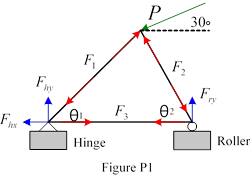P1:      Use MATLAB to solve linear simultaneous equations (Estimate time

required, 20 minutes.)

Consider the statically determinate truss shown in Fig.P1. The applied force has a magnitude of P=950(1+0.2S) N at an angle of 30° from the horizontal. The inner angles, θ1 and θ2 are 45° and 65° respectively.    The      following         linear   simultaneous equations can be used to solve for 6 unknown forces F1, F2, F3, Fhx, Fhy, Fry.

 −Pcos30°+ F1 cosθ1 − F2 cosθ2 = 0                        −F1sinθ1 + Fhy = 0      −Psin30°+ F1sinθ1 + F2 sinθ2 = 0                          F2 cosθ2 − =F3  0        −F1cosθ1 + F3 − Fhx = 0                                           −F2 sinθ2 + =Fry  0 P1.1 Convert this set of linear simultaneous equations into matrix format (hand solution). P1.2 Use suitable MATLAB commands to solve for 6 unknown forces F1, F2, F3, Fhx, Fhy, Fry.

Note that:  The parameter S in the applied force P is a parameter related to each student’s student id and this parameter must be generated before you start to solve this problem using the following MATLAB codes.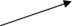RandStream.setGlobalStream(RandStream('mt19937ar','seed',1406833));

S=rand(1);

replace it with your student id

P1.3     Present the final results on command window using formatted text.

 What to present on the report? •      Hand solution of P1.1. •      Results of P1.3 (screen capture) •      MATLAB script. What to submit on CD? • Single MATLAB script file (program).

Ideas for solving this problem. P1.1

 −Pcos30°+F1cosθ1 −F2 cosθ2 = 0→⎡ cosd(45) −Psin30°+F1sinθ1 +F2 sinθ2 = 0 ⎢⎢ ??−F1cosθ1 +F F3 − hx = 0 ⎢ ?? −F1sinθ1 +Fhy = 0 ⎢⎢−sind(45)F2 cosθ2 −F3 = 0 ⎢ ?? −F2 sinθ2 +Fry = 0 ⎢⎣ ?? −cosd(65) ????0???? 0????0???? 0????0???? 0????1???? 0 ⎤⎡F1 ⎤ ⎡Pcosd(30)⎤ ??⎥⎢⎢F2 ⎥⎥ ⎢⎢ ⎥??⎥⎢F3 ⎥ ⎢0 ⎥⎥⎢⎢Fhx ⎥⎥=⎢⎢??⎥⎢Fhy ⎥ ⎢⎥⎢ ⎥ ⎢ ??⎦⎢⎣Fry ⎥⎦ ⎣ ????0???? ⎥⎥ ⎥ ⎥⎥ ⎥⎥ ⎦ P1.2:   Solve system of equations, See Lecture Notes page 13, Slides 50, 51.

P1.3:   To present formatted texts using command  fprintf, See Lecture Notes page 37, Slides 145, 146.

P2: Use MATLAB to solve constraint optimization problems in engineering design (Estimate time required 30 minutes)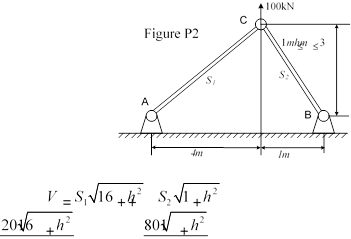Consider the two-bar truss shown in Figure P2. The objective is to minimize the volume V of the two bars AC and BC. The cross-sectional areas of the bars AC and BC are denoted as S1 and S2 respectively, and the vertical position of joint is denoted as h. The constraints of the system are that the tensile stresses on the two bars must be less than or equal to σ=105kPa and that the vertical position remains between 1m and 3m. Finally, S1 and S2 are nonnegative. (fmincon is the command for this type of question.)

The optimization objective is to minimize V

 hS1 ≤σ             ≤σ hS2 1≤h≤3 S1 ≥ 0                 S2 ≥ 0

subject to constraints:

Ideas for solving this problem.

The above objective function is a function of several variables. To solve this type of problem, the objective function must be first converted into a function of a single vector variable. The same should be done for constraint functions. Please see examples in Workshop 2 W2P3 and Lecture Notes Pages 26, 27, Slides 104, 105.

For the examples of solving constraint optimization, please see examples in Lecture Notes Pages 29‐31, slides 113‐122. Basically, you need to define TWO functions, one function for the cost (objective), another for describing constraints. Both functions can be defined as “in program” anonymous functions or by separate function files.

You need to do some preparation work to convert all constraints to the standard

format so that they can be described by the constraint function..

 What to present on the report? •      Any preparation work to convert the problem functions to standard format so that can be described in MATLAB. •      Final results (h, S1 and S2 which minimizes V and the minimal value for V). •      All MATLAB scripts (including function files if there are any). What to submit on CD? •      Main MATLAB script file (program). •      Two MATLAB function files if functions are defined as function files not anonymous functions.

P3: Curve Fitting/Model Identification: find a suitable formula/model to fit experiment data. (Estimate time required 45 minutes)

In a wind tunnel study of dense gas dispersion in a neutral boundary layer over a rough surface, the

z2

Gaussian function c = exp2s2 is used to model relationship amongst the average plume

concentration ratio (c), height (z) and vertical plume speed (s). The task is to find the parameter s for the above model using collected data. This is a curve fitting problem (find parameter s so that the above mathematical model fit the collected data).

 P3.1 Use MATLAB command xlsread to import data into z and c vectors from the data file WindTunnelData.xlsx (downloadable from Blackboard).   •       To find out how to use this command and examples, use    >> doc xlsread  •       Import data into a matrix, the 1st column is z and 2nd column is c. For handling specific matrix column (row), see Workshop 1 W1P1. P3.2 Curve Fitting (Model Identification) Method 1: The linear least square method can be z2 ⎞ ⎛ used to find the model parameter s so that the model (c = exp⎜⎝−2s2 ⎟⎠ ) fit experiment data (z, c vectors), the estimate value for s is kept by the variable s1.

Convert a nonlinear model into a linear model so that the linear least square method can be used

Nonlinear equations such as the one we have in here must be first converted into linear equations

c = exp⎜⎛2sz22 ⎠⎟⎞ ⇒ ln( )c =−21s2 z2

By defining     y= ln( ),c       x z and a= 2       =−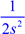The nonlinear equation has been converted to the linear equation              y = ax

The least square method is first used to estimate the parameter a. Once a is estimated, the corresponding estimate for s can be determined.

Linear Least Curve Fitting Method

For a linear equation y=ax and experiment data set (x(1), y(1)), (x(2), y(2)), …, (x(N), y(N)), the least

square estimation for a is given by

Naˆ =k=N1 x k y k( ( ))( ) ( ) = x y(1) (1)( (1))x +2 +x(2) (2)( (2))x y 2 + ++ +"" ( (x N y Nx N( ) ())2 ) sum x y sum x( .* ) / ( .^ 2) x k 2

k=1

Program steps

(1)    Calculate x and y according to the definition above.

In theory, the values for c should all be positive. However, some experiment values collected become negative because “measurement noise”. This may cause problem for the calculation of ln(c). This problem can be overcome by using ln(|c|) instead of ln(c).     log(abs(c)) in MATLAB.

(2)    Find the estimation of a using the least square formula.

(3)    Determine s using a.  (use the variable s1 to store the value of s in order to differentiate with the following parts).

 P3.3 Curve Fitting (Model Identification) Method 2: The nonlinear curve fitting problem can be solved by the MATLAB’s nonlinear curve fitting routine lsqcurvefit. Use this MATLAB routine to solve the curve fitting problem, i.e. find optimal parameter s and keep it in the variable s2. •     For nonlinear curve fitting example, see Lecture Notes Pages 50 & 51, Slides 197‐201. P3.4 Curve Fitting (Model Identification) Method 3: Due to a special property of the Gaussian function, the following relation can be used to find the value of s using experiment data.

s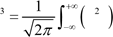z c dz

 Use “trapz( )” command to calculate the integration, the estimate (s3) of the parameter s is then determined using above formula. (The integration range from ‐∞ to +∞ simply means that the integration is for the whole range of z.) P3.5 Curve Fitting (Model Identification) Method 4: The model identification problem is an optimization problem which is to find the optimal parameters to make the sum of square of prediction errors minimum. Use a MATLAB’s optimization command (e.g. “fminsearch”) to find the parameter s  to minimize the following cost function. 2                           301⎛                  ⎛−z k( )2 ⎞⎞ J =∑= ⎜⎜c k( )−exp⎜2s2 ⎟⎠⎟⎟⎠             J is a function of s. k 1⎝     ⎝The estimate (s4) of the parameter s is determined by minimizing the above cost function. •       For minimization see Workshop 2 W2P1 & W2P2, Lecture Notes Page 25, Slide 98 •       The sum in the above function can be done in the following three ways                 →           sum( (c – exp(‐z.^2/?? )).^2)                 →           (c – exp(‐z.^2/?? ))’*(c – exp(‐z.^2/?? ))                 →           Loop, see example in Lecture Notes Page 45, Slide 179. P3.6 Present the results of all four methods on the screen using formatted text as follow. Method 1: the estimation of s is ??? Method 2: the estimation of s is ??? Method 3: the estimation of s is ??? Method 4: the estimation of s is ??? Present formatted texts using command  fprintf, See Lecture Notes page 37, Slides 145, 146. P3.7 To check the goodness of the models identified, the identified parameters (s1, s2, s3 and s4) will be used to determine the predicted model outputs according to the following equations. ⎛c1 = exp⎝⎜−z122 ⎟⎠⎞ c2 = exp⎜⎝⎛−2sz222 ⎟⎠⎞ c3 = exp⎛⎜⎝−2sz322 ⎞⎟⎠ c4 = exp⎛⎜⎝−2sz422 ⎞⎟⎠ 2s

Use plot command to plot five graphs (c versus z, c1 versus z, c2 versus z, c3 versus z, c4 versus z) on the same figure window.

plot(z, c, z, c1, ….)

 What to present on the report? •      P3.6: Results screen capture. •      P3.7: Plot.  •      All MATLAB scripts (including function files if there are any). What to submit on CD? •      Main MATLAB script file (program). •      MATLAB function files if functions are defined as function files not anonymous functions.

P4: Use MATLAB to solve complex engineering problem (Function files, program branches, conditional and logic operations, solving differential equations, numerical integration, 2-d plots) (Estimate time required 30 minutes)

Fig. P4.1 represents the “quarter-car” model of a car suspension system. The car travels at a constant horizontal velocity VH and the road surface has a chuck hole and a bump shown in Fig.P4.2. The equations for describing the dynamics of this system can be derived as

 ⎧y = vy⎪z = vz                         ⎨⎪Mvy = K2(z − y)+ B v( z −vy)⎪ ⎪mvz = K u1( − −z)    K2(z − y)− B v( z −vy) ⎩ ⎡ y ⎤dotx =⎢⎢ z ⎥⎥ ⎢vy ⎥⎢ ⎥ ⎣vz ⎦ ⎡ ⎤y⎢ ⎥zx =⎢ ⎥ ⎢ ⎥vy⎢ ⎥ ⎣ ⎦vz

displacement y(t)                               M=350kg:  mass of quarter car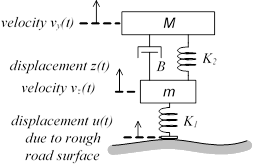K2:  suspension spring constant B:  shock absorber damping coefficient m=21kg:  unsprung tire mass

K1:  tyre compliance spring constant

Figure P4.1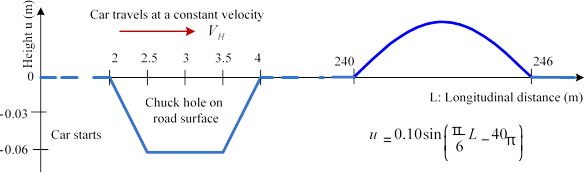Figure P4.2

P4.1 The first step to solve differential equations is to create a function to describe derivative on the right hand side of differential equations. Write a MATLAB function m-file to describe the dynamic equations with dotx as the function output. In addition to usual inputs of time (t) and x as inputs, the variable parameters VH, B, K1 and K2 will be used as input variables as well

The structure of this function will be

function dotx=funcname(t, x, VH, B, K1, K2)

M=350; m=21; L=VH*t; if L>=0 & L<2     u=0; elseif L>=2 & L<2.5     u=(-0.06/0.5)*(L-2)

????????     ???????     ????????

end y=x(1);z=x(2);vy=x(3);vz=? dotx=[vy;?;(K2/M)*(z-y)+??? ; ???]; end

To test the correctness of this function, try the following to produce a 4x1 vector

>> funcname(3, [1;2;0;3], 19, 1100,20000, 10000)

P4.2 Given parameters B=1140Ns/m, K2=20900N/m and K1=10800N/m, VH=60mph (convert to m/s), use a MATLAB differential equation solver (ode15s, ode23s or ode23t) to simulate the response of the car suspension system for the time period [0, 20] seconds. The initial conditions are all as zeros.

Examples of solving multiple 1st order differential equations, see Workshop 2 W2P4 and Lecture Notes pages 33 & 34 Slides 129‐135.

For solving differential equations with additional parameters, see Lecture Notes pages 34 & 35 Slides 136 & 137.

The ODE solver ode45 will NOT produce correct results for this problem, therefore ode15s, ode23s or ode23t will be used. General the ODE solver can automatically determine the best step size to use to solve ODEs. However, for this particular problem, the maximum step size must be specified to get a solution. See Below  VERY IMPORTANT.

 options=odeset('MaxStep',0.1); [T,X]=ode23s(@(t,x)funcname(t,x,VH,B,K1,K2),[0  20],[????],options);

(a)               Plot y, z, vy, vz versus t in 4 subplots.

o   y, z, vy, vz can be picked out from X, i.e.  y=X(:,1),..., vz=X(:,4)o For using subplot command, see Lecture Notes page 19 Slides 74 & 75.

(b)               The car’s passenger comfort can be evaluated using the car body’s displacement (y) and vertical acceleration (a = vy ). From simulation results, determine and plot the vertical acceleration (a = vy ) and displacement (y) all against the time t. Find the maximal vertical displacement and the root mean square of the acceleration

arms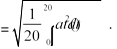o   The      vertical            acceleration    can       be        determined     using    the       formula,K2 (z − +y) B (vz vy). ay = =vy M M

o   The command trapz(t, ay) can be used to calculate the integration.

 What to present on the report? •      P4.2, plots, results for vertical displacement and acceleration root mean square. •      All MATLAB scripts (including function file). What to submit on CD? •      Main MATLAB script file (program). •      MATLAB function file.

P5: Use MATLAB to solve complex engineering problems (functions, differential equation solutions, data interpretation, loop, conditional and logic operations, combining arrays, 2-d plots and subplots) (Estimate time required 90 minutes)

A ball is thrown into the air with the initial velocity v0 and the angle α to horizontal direction (see Figure P5a). The air is assumed to have friction which is proportional to the velocity. The horizontal and vertical velocities and positions can be determined by solving the following differential equations (derived from the Newton’s law).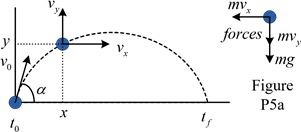⎧⎪dvdtx =− mB vx

⎪⎪dvdty =− −gmB vydx = vx dt dy= vy

dt

 ⎧vx(0) = v0 cosα⎪vy(0) = v0 sinα                        ⎪⎨                         x(0)                                 ⎪        = 0⎪⎩y(0) = 0 α= 36 (1° +0.25 )Sv0 = 29(1+0.24 )Sm =10                       B = 2 g           9.81

=

Note that S is the student related parameter generated in the same way as P1.

P5.1 Use a differential equation solver in MATLAB (i.e. ode45, ode23 or ode15s) to solve the above equations to find the solution for vx, vy, x, y and corresponding time history t for the time period of [0, 6] seconds. Use subplot command to plot four graphs (vx versus t, vy versus t, x versus t, y versus t) on the same figure window. (Don’t present all date of vx, vy, x and y on command window screen and the Report. Only graphs need to be presented on the report.)

Examples of solving multiple 1st order differential equations, see Workshop 2 W2P4 and Lecture Notes pages 33 & 34 Slides 129‐135.

The first step is to define a MATLAB function (anonymous function or function file) to describe the ball throw dynamic equations with dotz as the function output, t (time) and z as the input. Note that the only task for this function is to describe four differential equations in vector format, nothing else should be put into this function!!

dvx

dt ⎥     dotz(1)                    vxz(1)

⎢      ⎥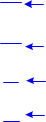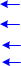dvy ⎥     ⎢vy⎥⎥z(2) dotz =⎢⎢ dt ⎥⎥    dotz(2) z =⎢⎢ x z(3) dx ⎥       dotz(3) y z(4)

dt

dy ⎥     dotz(4)

⎢      ⎥

dt ⎦

Before you move to next step, it is a good idea to test this function,

>>funcname(2, [1  2  3  4]) should produce a 4x1 column vector.

How to use ODE solver?

[th, zh]=ode54(@funcname, [0 6], initial condition vector z0).

Here, th is the history of time t for the period of [0  6], vx, vy, x, y can be picked out from zh, i.e.  vxh=z(:,1)..., yh=z(:,4)

For using subplot command, see Lecture Notes page 19 Slides 74 & 75.

P5.2 Find the time (tf) when the ball strikes the ground, i.e. y=0. The way to find the time tf is to simulate (solve) the system differential equations for a longer period time (done in P5.1) and then use “interp1” to find tf when y(tf)=0.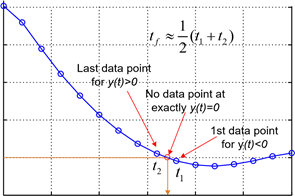This can be done by the find command (Lecture Notes page 47) and/or the interpretation (Lecture Notes page 49 Slides 189 & 190). From the graph on the right, it can be seen that no data point at exactly y(t)=0, so that some approximations must be made. We can find the first data point when y(t)<0 and the last data point when y(t)>0 and then approximately determine tf.

Method 1: Very rough approximation

Pindex=find(y<0); tf=(t(Pindex(1))+t(Pindex(1)-1))/2; 0

Method 2: Approximate with more data points

Pindex=find(y<0);

yselect=y(Pindex(1)-3:Pindex(1)+3); 0 Time (seconds)t f tselect=t(Pindex(1)-3:Pindex(1)+3); tf=interp1(yselect, tselect,0);

 P5.3 For the time interval [0, tf], repeat the ODE solution of P5.1 to calculate the complete time history of t, vx, vy, x and y. Use subplot command to plot four graphs (vx versus t, vy versus t, x versus t, y versus t) on the same figure window. (Simply replace [0 6] in P5.1 with P5.2) P5.4 From Fig.P5b, it can be seen that the ball bounces back into the air when it strikes ground. It is assumed that the ball bounces back with a reduced projection angle α and a reduced velocity according to the following reduction rate. v1 = v0 ,                                                 α1 =α v2 = 0.7(1+0.21 )S v1,                           α2 =α1 −2° v3 = 0.7(1+0.21 )S v2 ,                         α3 =α2 −2° ………… Find out how many bounces the ball will complete before its bouncing back velocity is less than the half of the first projection velocity.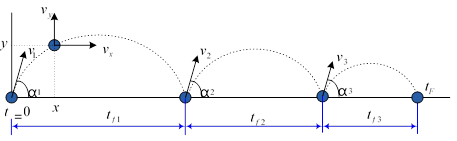Figure P5b

Note:    while-end loop is used to complete this task, but for-end loop can also be used

V(1)=v0;     %Vector V to store all bounce velocities v1, v2, .....

Alpha(1)=??; %Vector Alpha to store bounce angles alpha1, alpha2, ..... bn=1;        % The number of bounce

fprintf(' ')

fprintf('Original projection velocity and angle are %g and %g ',v0,alpha0)

fprintf('The original projection velocity is %g ',v0)

while (V(bn) >= 0.5*v0) & (bn <100)  %Restrict maximum loop number to avoid infinite loop     bn=bn+1;

V(bn)=???*V(bn-1);

Alpha(bn)=????;

fprintf('The velocity and angle of bounce %g is %g and %g ',bn-1,V(bn),Alpha(bn)) end

fprintf('Bounce number before bounce velocity less than 0.5 of original velocity is:   %g ',bn-1)

 P5.5 For each projection (original and subsequent bounces), find the time when the ball hits ground and then calculate the complete time history of t, vx, vy, x and y. (Do not present all data values on the command window and the report. Those data will be used in the following task for plotting). •       Basically, you just need to repeat the tasks P5.1, P5.2 and P5.3 for every projection (without plots). •       You could put the tasks performed in P5.1, P5.2 & P5.3 (No plots) into an function file, i.e. write a function to calculate tf and the history of t, vx, vy, x and y (outputs) based on v0 and α (inputs) (function [tf, th, vxh, vyh, xh, yh] = p5funRep(alpha,v0)). The data for each bounce can then be determined using this function, i.e  [t1 tf1 x1 y1 vx1 vy1]= p5funRep(v0(1),Alpha(1)) [t2 tf2 x2 y2 vx2 vy2]= p5funRep(v0(2),Alpha(2)) ……………………………………………………………………… P5.6 Put data for all projections together to produce one figure to plot the ball’s whole trajectory (y vs x) (this plot should be similar to Figure P5b), and another figure which has 4 subplots (vx vs t, yy vs t, x vs t and y vs t, all for the time period [0, tF]). Hint:    The histories of all projections can be combined together as following

T=[t1; t2+tf1; t3+tf1+tf2;.............];

X=[x1; x2+x1(end); x3+x2(end)+x1(end) .................];

Y=[y1; y2; y3;..............];

VX=[ vx1; vx2; vx3......];

VY=[ vy1; vy2; vy3.......];

NOTE: The commands given above combine COLUMN vectors together. To combine row vectors together, replace ; with ,  e.g. Y=[y1, y2, y3,..............]

 What to present on the report? •      P5.1 plots. •      P5.2 result (screen capture). •      P5.3 plots •      P5.4 result (screen capture). •      P5.6 plots •      All MATLAB scripts (including function files). What to submit on CD? •      Main       MATLAB          script    file (program). •      MATLAB function files if functions are defined as function files not anonymous functions.

P6 MATLAB program branches (Estimate time required 20 minutes, example see Workshop 3 W3P4 )

Write a MATLAB program to call all programs written for all problems for this assignment.

When this program is run, a selection menu similar to the following one should be presented.

Select Problem to solve:

1: Problem P1

2: Problem P2

……………………………………

5: Problem 5

Once the selection is made, the relevant program should be executed to solve the problem selected. (Use MATLAB command “switch-case-end”). The program should be able to return to the selection menu before all problems have been solved.

 What to present on the report? • All MATLAB scripts. What to submit on CD? • MATLAB script file (program).

Is it free to get my assignment evaluated?

Yes. No hidden fees. You pay for the solution only, and all the explanations about how to run it are included in the price. It takes up to 24 hours to get a quote from an expert. In some cases, we can help you faster if an expert is available, but you should always order in advance to avoid the risks. You can place a new order here.

How much does it cost?

The cost depends on many factors: how far away the deadline is, how hard/big the task is, if it is code only or a report, etc. We try to give rough estimates here, but it is just for orientation (in USD):

 Regular homework \$20 - \$150 Advanced homework \$100 - \$300 Group project or a report \$200 - \$500 Mid-term or final project \$200 - \$800 Live exam help \$100 - \$300 Full thesis \$1000 - \$3000

How do I pay?

Credit card or PayPal. You don't need to create/have a Payal account in order to pay by a credit card. Paypal offers you "buyer's protection" in case of any issues.

Why do I need to pay in advance?

We have no way to request money after we send you the solution. PayPal works as a middleman, which protects you in case of any disputes, so you should feel safe paying using PayPal.

Do you do essays?

No, unless it is a data analysis essay or report. This is because essays are very personal and it is easy to see when they are written by another person. This is not the case with math and programming.

Why there are no discounts?

It is because we don't want to lie - in such services no discount can be set in advance because we set the price knowing that there is a discount. For example, if we wanted to ask for \$100, we could tell that the price is \$200 and because you are special, we can do a 50% discount. It is the way all scam websites operate. We set honest prices instead, so there is no need for fake discounts.

Do you do live tutoring?

No, it is simply not how we operate. How often do you meet a great programmer who is also a great speaker? Rarely. It is why we encourage our experts to write down explanations instead of having a live call. It is often enough to get you started - analyzing and running the solutions is a big part of learning.

What happens if I am not satisfied with the solution?

Another expert will review the task, and if your claim is reasonable - we refund the payment and often block the freelancer from our platform. Because we are so harsh with our experts - the ones working with us are very trustworthy to deliver high-quality assignment solutions on time.

Customer Feedback

"Thanks for explanations after the assignment was already completed... Emily is such a nice tutor! "

Order #13073